# Using the equation A + B --> C + D, answer the following questions about the...

Using the equation A + B --> C + D, answer the following questions about the rate equation for this reaction.

.

. If this reaction is bimolecular, there are three possible rate equations. Write them.

rate 1 =

rate 2 =

rate 3 =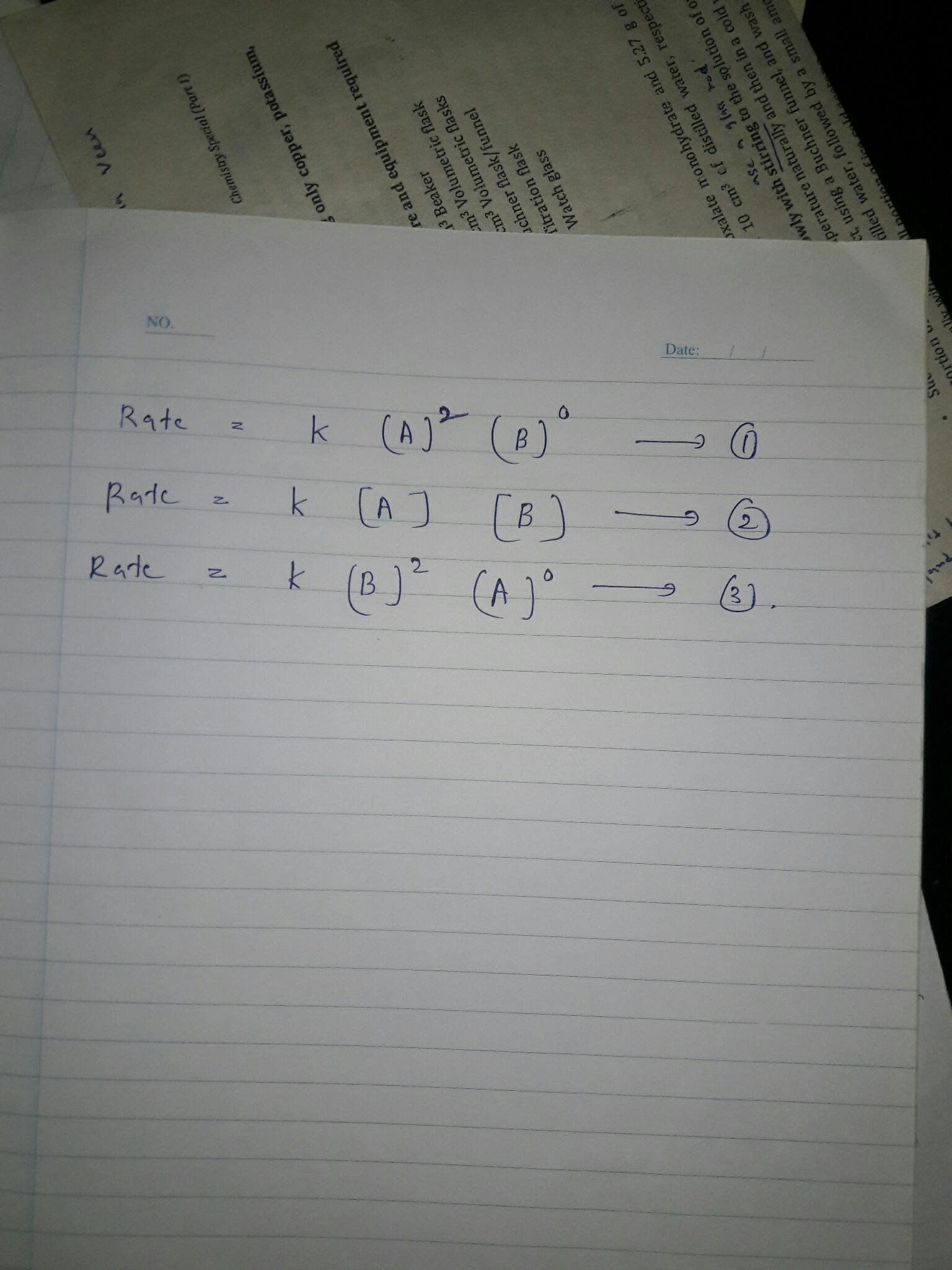#### Earn Coin

Coins can be redeemed for fabulous gifts.

Similar Homework Help Questions
• ### Answer the following questions about inflation: a) What is the generic inflation equation? b) What does...

Answer the following questions about inflation: a) What is the generic inflation equation? b) What does Friedman add to it? c) Who is inflation good for? Who is it bad for? d) Suppose inflation expectations are 3%, the employment gap is 2%, and supply shocks are 3%. What would the inflation rate be?

• ### 18 pts] Use the following equation to answer the following questions: F = a' + ac'...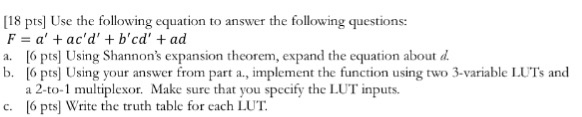18 pts] Use the following equation to answer the following questions: F = a' + ac' d' + b' cd' + ad a. 6 pts Using Shannon's expansion theorem, expand the equation about d b. 6 pts Using your answer from part a., implement the function using two 3-variable LU'T's and Make sure that you specify the LUT inputs. a 2-to-1 multiplexor. c. 6 pts] Write the truth table for each LUT

• ### this is a pre lab about rate of reaction i need help with the three questions...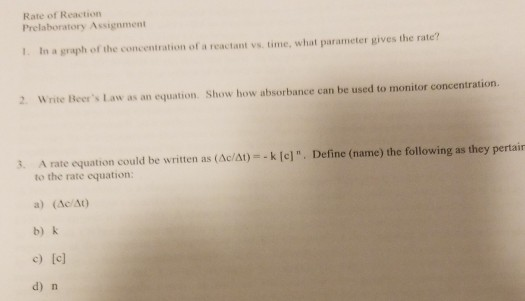this is a pre lab about rate of reaction i need help with the three questions Rate of Reaction Prelaboratory Assignment 1. In a graph of the concentration of a reactant vs time, what parameter gives the rate? 2. Write Beer's Law as an equation. Show how absorbance can be used to monitor concentration 3. A rate equation could be written as (Ac/At)- k [e]". Define (name) the following as they pertair to the rate equation: a) (Ac/At) b) k...

• ### Done Problem 1. Consider the general reaction 2A+2B+C D+3E And answer the following questions usi...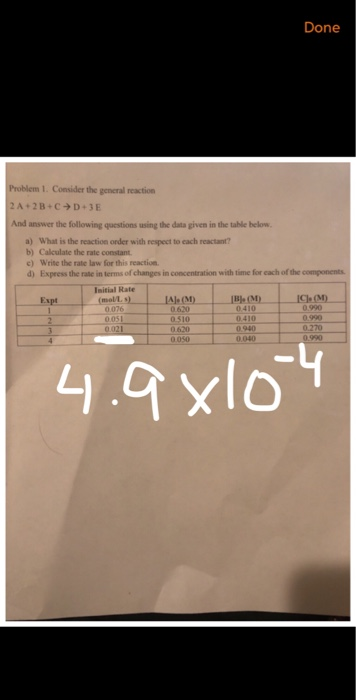Done Problem 1. Consider the general reaction 2A+2B+C D+3E And answer the following questions using the data given in the table below. a) What is the reaction order with respect to each reactant? b) Calculate the rate constant c) write the rate law for this reaction. d) Express the rate in terms of changes in concentration with time for each of the components Initial Rate 1 0180:101 076 05 0.990 0.990 0.270 0.620 0.620 0050 0410 0.940 0.040 Done Problem...

• ### Answer the following questions using the balanced chemical equation below: Bi2O3 + 3 C ? 2...

Answer the following questions using the balanced chemical equation below: Bi2O3 + 3 C ? 2 Bi + 3 CO a. What is the limiting reactant when 232.47 g Bi2O3 reacts with 27.03 g C? b. What is the mass of bismuth produced from this reaction (i.e. the theoretical yield)? c. If the actual yield is 123.20 g Bi, what is the percent yield? d. How much excess reagent is left?

• ### 2. Answer the following questions about phosphoric acid (H3PO4). a. (2 points) What color will a...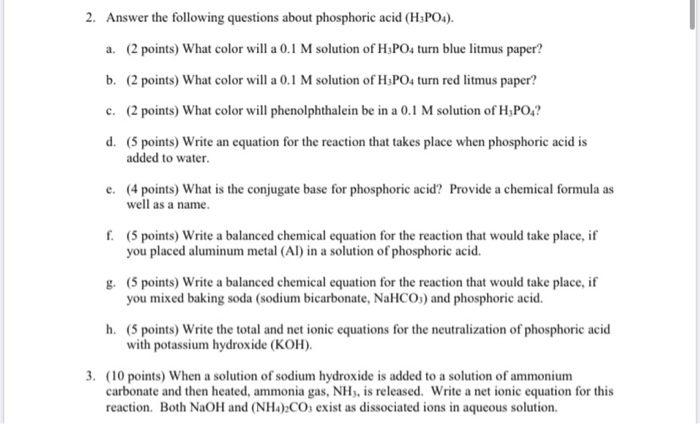2. Answer the following questions about phosphoric acid (H3PO4). a. (2 points) What color will a 0.1 M solution of H3PO4 turn blue litmus paper? b. (2 points) What color will a 0.1 M solution of H3PO4 turn red litmus paper? c. (2 points) What color will phenolphthalein be in a 0.1 M solution of H,POA? d. (5 points) Write an equation for the reaction that takes place when phosphoric acid is added to water e. (4 points) What is...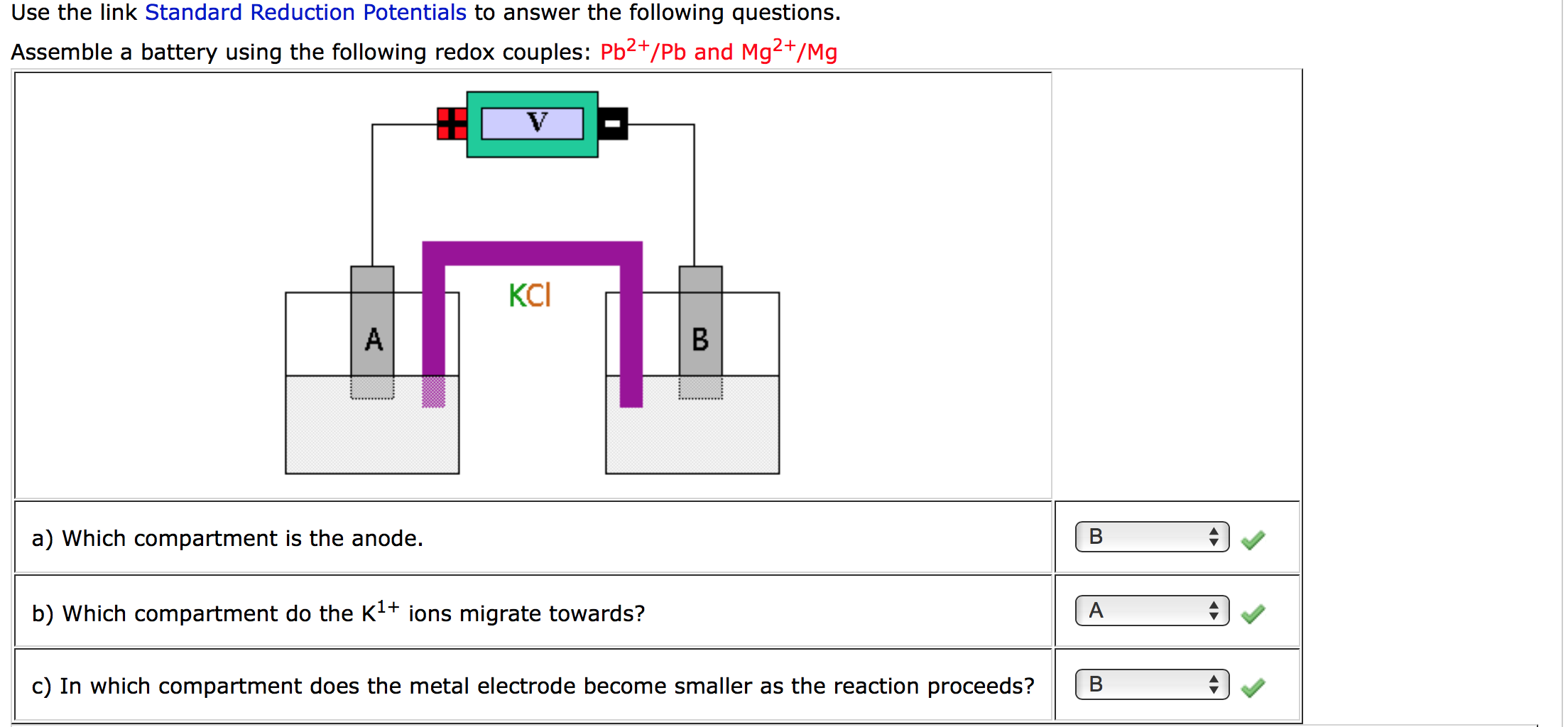Please help with parts d-f Use the link Standard Reduction Potentials to answer the following questions. Assemble a battery using the following redox couples: Pb2+/Pb and Mg2+/Mg a) Which compartment is the anode. b) Which compartment do the K1+ ions migrate towards? LA A c) In which compartment does the metal electrode become smaller as the reaction proceeds? Help chemPad X.X" Pb2+ + 2e + Pb Pb^2+ + 2e^- --> Pb Greeka d) Write the half reaction that occurs at...

• ### The Beer's law equation parameters mean, A for abosrbance, c for conenctration (mol/L), b for the...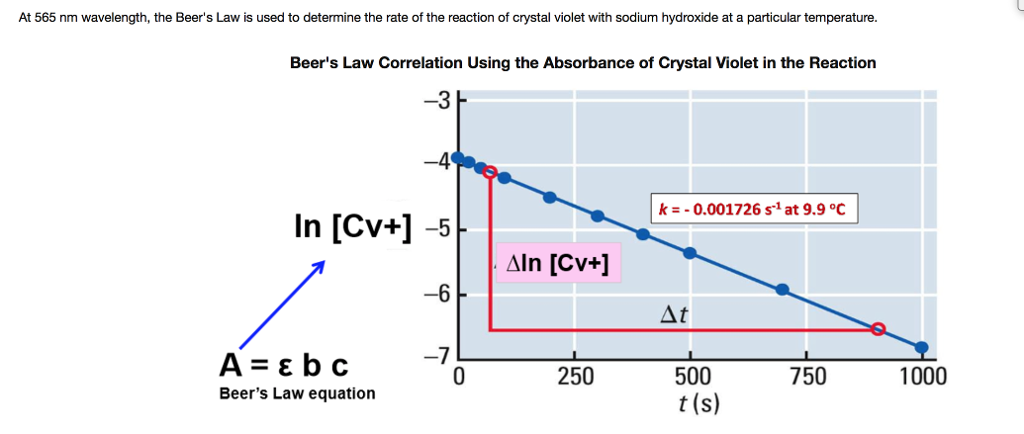The Beer's law equation parameters mean, A for abosrbance, c for conenctration (mol/L), b for the pathh of light (cm), and E for absoprtivity constance. Using the graph above, answer the following questions: 1. Briefly explain the relationship between the concentration and absorbance of crystal violet on the graph 2. Based on teh shape of the graph, write the applicable integrated rate law for the reaction 3. What is the rate constant of the reaction? 4. Write the complete rate...

• ### Please answer all parts to this question! parts a through d with the explanation on question...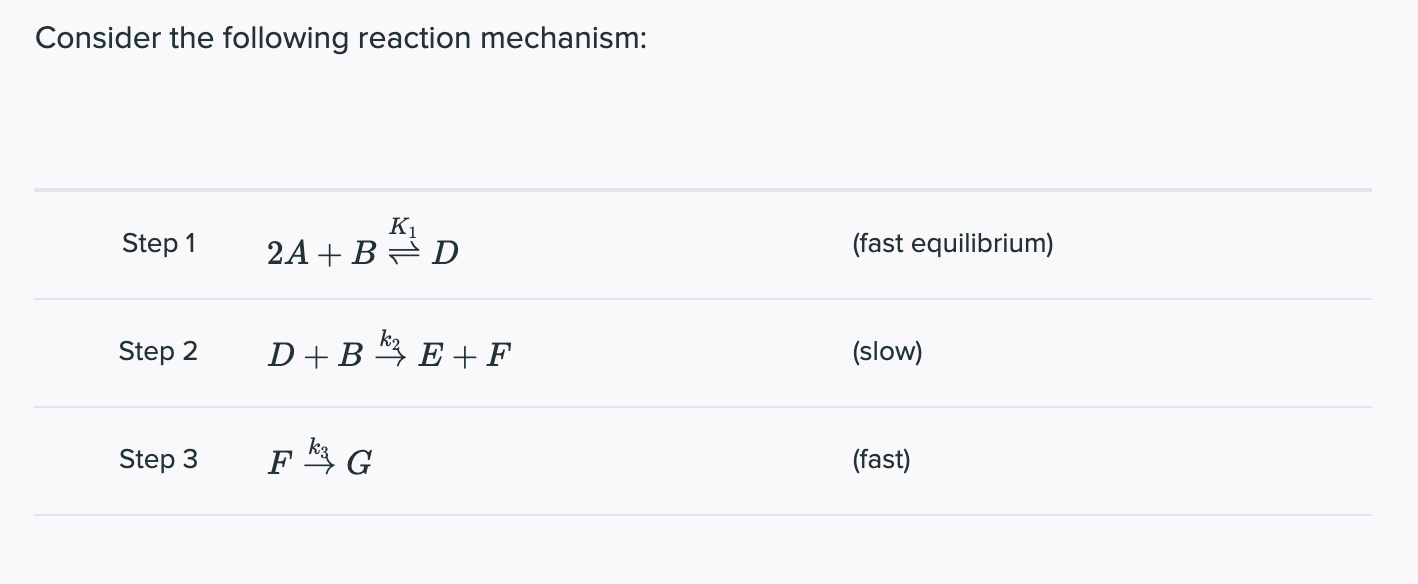Please answer all parts to this question! parts a through d with the explanation on question d! This is all one question so I could not post them separately, please also check your answers because the last person got it wrong! Thank you! WIll give thumbs up. Consider the following reaction mechanism: Step 1 K 2A + B =D (fast equilibrium) Step 2 D+B k? E+ F (slow) Step 3 F G (fast) a. Give the molecularity of each step....

• ### please answer all questions for rate. thank you :) For the following equation: MnO4 (aq) +...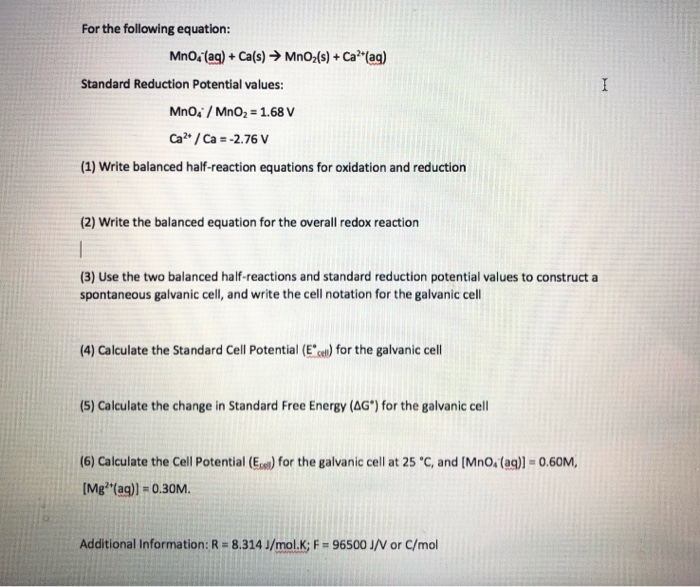please answer all questions for rate. thank you :) For the following equation: MnO4 (aq) + Ca(s) → MnO2(s) + Ca?*(aq) Standard Reduction Potential values: Mno. MnO2 = 1.68 V Ca? / Ca = -2.76 V (1) Write balanced half-reaction equations for oxidation and reduction (2) Write the balanced equation for the overall redox reaction (3) Use the two balanced half-reactions and standard reduction potential values to construct a spontaneous galvanic cell, and write the cell notation for the galvanic...